# AutoPhysics Settings

AutoPhysics is a complex tool. Its behavior can be tuned within wide limits using a relatively large set of parameters.

For convenience, these parameters are separated into several tabs that can be found on the Physics Settings panel.

## Basic Settings

This group controls the main features of the Physics Ghosts. It is available on the AutoPhysics tab: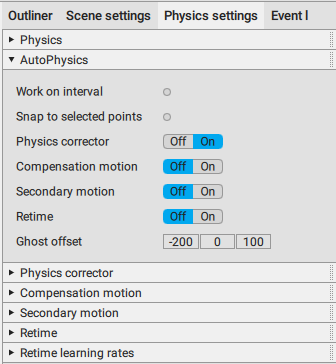Work on interval
If this is enabled, AutoPhysics simulation is applied only to the selected interval.
Otherwise, it is applied to the entirety of the Timeline.
Disabled by default.

Snap to selected points
If this option is enabled, the animation is snapped to the Auto Physics only for the selected Point Controllers. Other controllers are not affected.
Disabled by default.

Fulcrum Area Indent
How much the fulcrum point is increased.
Used in situations when the corresponding parts of the character mesh happen to be bigger than the “default” size of the fulcrum point(s) calculated on the basis of the Point Controllers.

The default value is 5.

Vertical Force Smoothness
This parameter defines how much the vertical (the Y axis) movement of the character is smoothed for the AutoPhysics simulation. The higher this value is, the more linear the trajectory will be.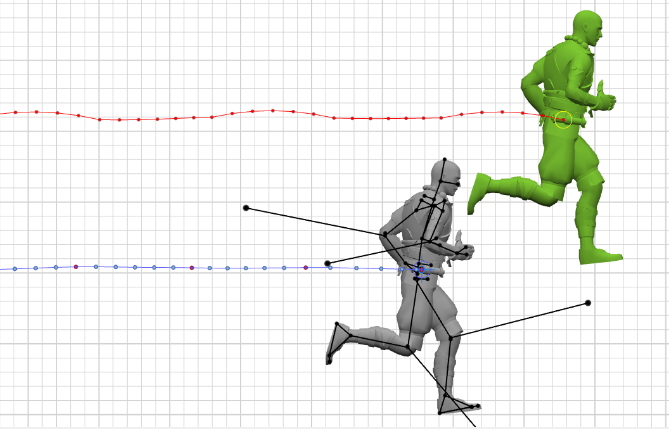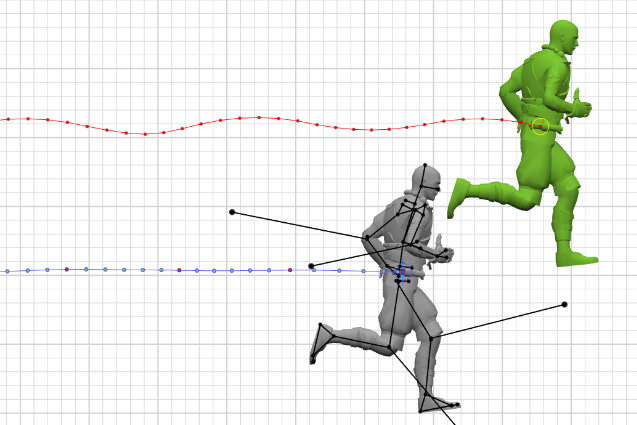The same animation with Vertical Force Smoothing set to 50 (up) and to 200 (down).

Set to 20 by default.

Ang. Momentum Smoothness
This setting defines how much the tool smoothes the character’s rotation. The higher its value, the more the character’s poses will be altered to remove distortions of the Angular Momentum value.

The default value is 50.

Ghost offset
These are the three coordinates of the physical assistant rendered in the Viewport window.
The coordinates work in relation to the character’s position, i.e. if all three are set to zero, the position of the physical ghost would coincide with that of the character.

This value can also be adjusted right in the Viewport window.

1. Left-click the assistant to select it.

2. Use the Translate manipulator.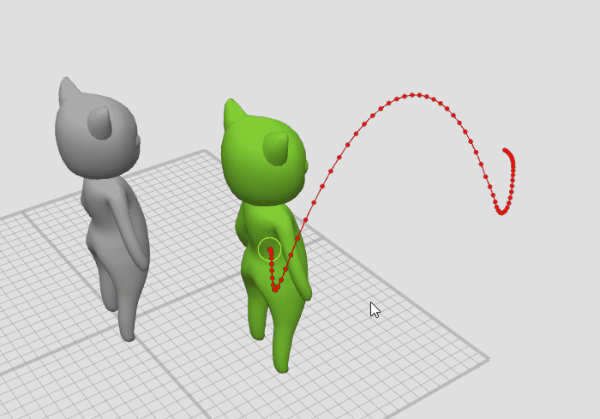Full cycles
Defines how many times the tool should simulate snapping the character to the AutoPhysics ghost.
Increasing the number of cycles improves the accuracy of the calculation. However, this also slows the tool down.

This parameter can be either set manually or using one of the pre-defined values (1, 2 and 5). The default value is 2.

Auxiliary settings for AutoPhysics can be found in the Settings Window, under the AutoPhysics tab: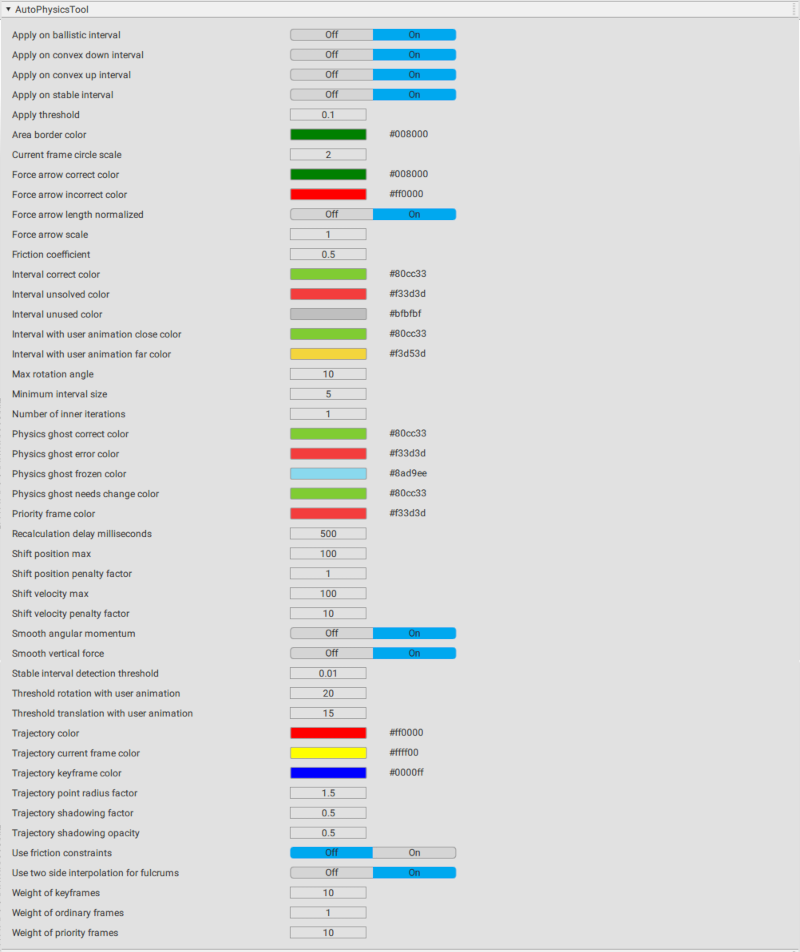Apply on ballistic interval
If this is enabled, the simulation is applied to the parts of the trajectory that are considered ballistic.
Enabled by default.

Apply on convex down interval
If this is enabled, the simulation is applied to the parts of the trajectory that have a convex shape facing downward.
Enabled by default.

Apply on convex up interval
If this is enabled, the simulation is applied to the parts where the trajectory of the character’s center of mass has a convex (arching) shape facing upward.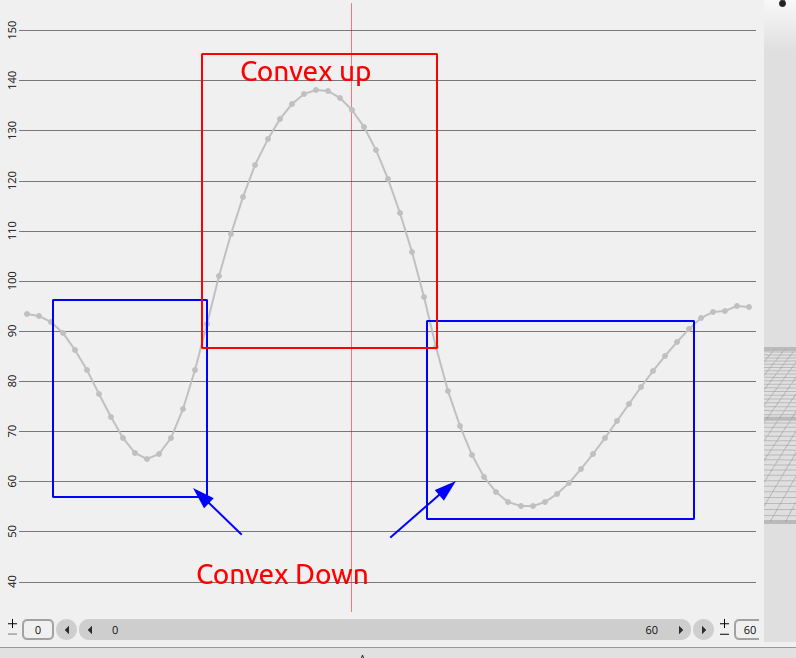Apply on stable interval
Defines if the tool should be applied on the stable (non-ballistic) intervals.
Enabled by default.

Apply threshold
If the distance between the current Center of Mass and its position suggested by the simulation is smaller than this value, the position does not change.
Measured in Cascadeur units (which are equal to centimeters).

Area border color
The color of the borders of the line representing AutoPhysics simulation on the Timeline.

Current frame circle scale
The scale factor for the circle that marks the current frame of the AutoPhysics trajectory

Force arrow correct color
The color of the force arrow when the simulation is correct.

Force arrow incorrect color
The color of the force arrow when the simulation is not correct.

Force arrow length normalized
If this is enabled, the length of the force arrow is normalized.
Enabled by default.

Force arrow scale
The multiplier for the length of the force arrow.
Set to 1 by default.

Friction coefficient
Defines the maximum value of the horizontal force applied to the character on the basis of the vertical force.
For example, if this value equals 0.25, the horizontal force cannot be greater than one quarter of the horizontal force.
Its default value is 0.5.

Interval correct color
The color used for marking the frames where there are no deviations from the AutoPhysics simulation.

Interval unsolved color
This color is used for marking the intervals where a physically accurate solution is not possible.

Interval unused color
This is the color for marking the intervals where AutoPhysics simulation is not calculated.

Interval with user animation close color
This color is used for marking intervals with user animation close to the AutoPhysics simulation.

Interval with user animation far color
This color is used for marking intervals with user animation far from AutoPhysics simulation.

Max rotation angle
The character cannot be rotated by an angle greater than this value.
Measured in degrees, set to 5 by default.

Minimum interval size
The minimal length (in frames) of the part to subdivide the animation into.
AutoPhysics works by separating the animation into small chunks and calculating how the character should move in every one of them.
The default value is 5.

Number of inner iterations
The number of regular cycles of physics simulation. Does not simulate snapping.
The default value is 1.

Physics ghost correct color
The color used for the physics ghost when there’s no abnormalities in the AutoPhysics simulation.

Physics ghost error color
The color used for the physics ghost where a correct solution cannot be found.

Physics ghost frozen color
The color used for the physics ghost when the simulation is frozen.

Physics ghost needs change color
The color used for the physics ghost when the simulation has to be recalculated.

Priority frame color
The color used for marking Physics priority frames for the AutoPhysics simulation.

Recalculation delay milliseconds
The delay between user-made changes to the animation and recalculating the simulation.
Measured in milliseconds. Set to 500 (0.5 seconds) by default.

Shift position max
This parameter limits how much the initial position can be shifted.
Measured in Cascadeur units.
Can be either set manually or using one of the predefined values (110 and 100). Its default value is 100.

Shift position penalty factor
This is the penalty for altering the initial position of the character (on the XZ plane).
This parameter can be either set manually or using one of the pre-defined values (110 and 100). Its default value is 1.

Shift velocity max
This parameter limits how much the character’s velocity can be shifted.
The value can be either set manually or using one of the predefined values (110 and 100). Its default value is 100.
Shift velocity is measured in Cascadeur units per frame. This means that scaling the scene up or down might prevent AutoPhysics from solving the given animation.

Shift velocity penalty factor
Same as above, but applied to the character’s initial velocity.
Its default value is 10.

Smooth angular momentum
If this is enabled, the tool will filtrate the character’s angular momentum.
Enabled by default.

Smooth vertical force
If this is enabled, the vertical (the Y axis) movement of the character is smoothed for the AutoPhysics simulation.

Stable interval detection threshold
The threshold used for determining whether an interval is stable or not.
By default, it is set to 0.01.

Threshold rotation with user animation
This value sets how much the character rotation in the user-created animation can differ from the AutoPhysics simulation without being considered physically inaccurate.
If the difference is less than this value, the corresponding part of the Timeline is colored green; otherwise, it is colored yellow.
Measured in Cascadeur units.
Set to 20 by default.

Threshold translation with user animation
Same as above, but for character translation.
Measured in Cascadeur units.
Set to 15 by default.

Trajectory color
The color used for rendering the trajectory of the physics ghost.

Trajectory current frame color
The color used for marking the current frame on the physics ghost trajectory.

Trajectory keyframe color
The color used for keyframes on the physics ghost trajectory.

Trajectory point radius factor
The value that controls the size of the point on the physics ghost trajectory.

Use friction constraints
If this is enabled, the horizontal force applied to the character is limited on the basis of the value of the vertical force.
Disabled by default.

Weight of keyframes
This value defines how much the character’s pose on a keyframe influences the AutoPhysics simulation.
Set to 10 by default.

Weight of ordinary frames
Same, but for regular frames.
Set to 1 by default.

Weight of priority frames.
Same, but for physics priority frames.
Set to 10 by default.